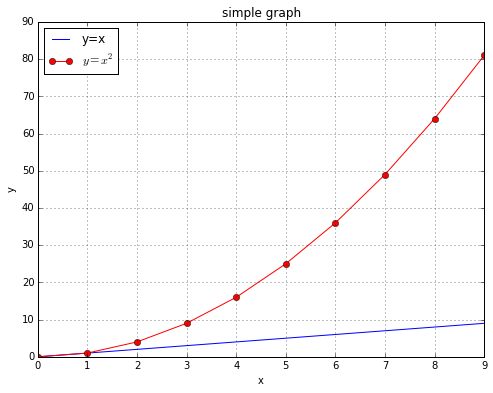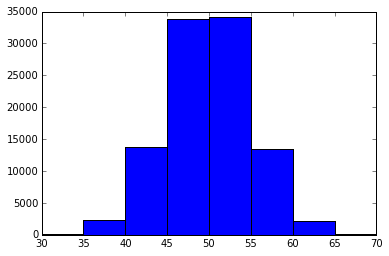In :
print("hello world")
hello world
In :
a='hell'
b='o, w'
c='orld'
print(a+b+c)
hello, world
In :
d=a+b+c
In :
print(d+'!')
hello, world!
In :
d,d,d[-1],d[3:6]
Out:
('h', 'l', 'd', 'lo,')
In :
# make graphics inline in notebook:
%matplotlib inline

import matplotlib.pyplot as plt   #plot routines in own namespace
import numpy as np                # numerical python
from numpy.random import rand     # generates random numbers between 0 and 1
In :
np.arange(10)**2
Out:
array([ 0,  1,  4,  9, 16, 25, 36, 49, 64, 81])
In :
plt.figure(figsize=(8,6))
plt.plot(np.arange(10))
plt.plot(np.arange(10)**2,'o-r')
plt.legend(['y=x','$y=x^2$'],loc='upper left')
plt.grid('on')
plt.xlabel('x')
plt.ylabel('y')
plt.title('simple graph');In :
plt.legend?
In :
rand(10) <.5
Out:
array([False,  True,  True, False,  True, False, False,  True, False,  True], dtype=bool)
In :
#see how many of 100 coin flips come up heads
#rand(N) is a list of 100 random numbers between 0 and 1
#sum() in this case counts the number of times the condition is True

sum([r < .5 for r in rand(100)])
Out:
59

stdev for this is sqrt(N*p(1-p))=sqrt(100/4) = 5, so by "68-96-99.7 rule":
roughly 68% of the time between 45 and 55
95.5% of the time between 40 and 60,
and 99.7% of the time between 35 and 65 .
Let's do T=100,000 trials to confirm, then draw a histogram:

In :
T=13000
data=[sum([r < .5 for r in rand(100)]) for t in xrange(T)]
for s in 5,10,15:
print '50 +/-',s,len([d for d in data if 50-s <= d < 50+s])/float(T)
50 +/- 5 0.679230769231
50 +/- 10 0.953538461538
50 +/- 15 0.997384615385
In :
len(data)
Out:
13000
In :
data[:10]
Out:
[49, 51, 49, 47, 46, 62, 44, 52, 51, 52]
In :
plt.hist(data,bins=np.arange(0,101,5))
plt.xlim(30,70);In [ ]:
#data +=  .1*(-1)**(rand(len(data)) > .5)
In [ ]: